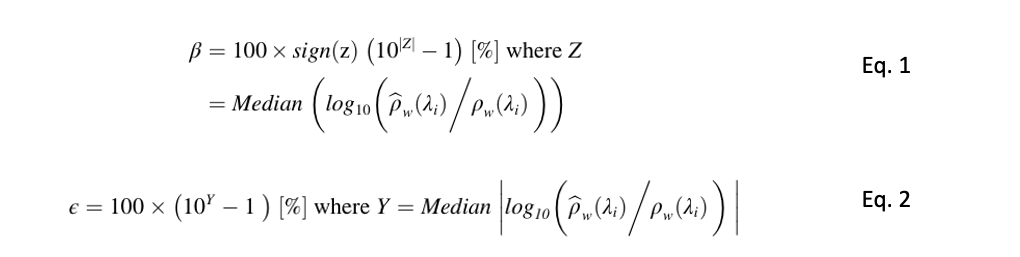## ACIX II Aqua Metrics

#### CONTACT

Metrics

We mainly focused on investigating two metrics representing the overall error and bias in target quantities. These two norms first transform data into log space and then convert them back to linear space to assess the quality of the retrieved quantity (e.g.,) against that measured in situ (e.g.,ρw). These metrics are computed as follows:where λi refers to spectral band i, Median is the median operator, β is the symmetric signed percentage bias and ε represents the median symmetric accuracy (MdSA; Morley et al. (2018)). These metrics, expressed in %, are simple for interpretation, reasonably resistant to outliers, and zero-centered compared to those in Seegers et al. (2018). In the definition of the above metrics, we assume no uncertainties in insitu measurements, because to a large extent these uncertainties affect all the AC processors equally and were unknown at the time of this research.

We also computed the Root Mean Squared Log Error (RMSLE), Root Mean Squared Error (RMSE), Median Absolute Percentage error (MAPE), slope of linear regression in log-space (S), and Mean Symmetric Accuracy (MSA) computed analogous to Eq. 2, except the average of the log ratios was employed to infer the overall impact of noisy retrievals (Seegers et al., 2018). Note that RMSE and MAPE are computed to merely provide traceability to previous studies (Pahlevan et al., 2017b; Pahlevan et al., 2017c; Steinmetz and Ramon, 2018; Warren et al., 2019) and is not anticipated to serve as a reliable measure given the log-normal distribution of our matchup datasets.